DraftKings Super Bowl Odds
+550
5.5 to 1Bills
13.4% implied probability

+600
6 to 1Buccaneers
12.5% implied probability

+700
7 to 1Chiefs
10.9% implied probability

+850
8.5 to 1Rams
9.2% implied probability

+1000
10 to 1Ravens
7.9% implied probability

+1100
11 to 1Cardinals
7.3% implied probability

+1200
12 to 1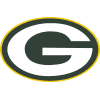Packers
6.7% implied probability

+1400
14 to 1Cowboys
5.8% implied probability

+1800
18 to 1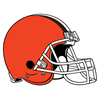Browns
4.6% implied probability

+2000
20 to 1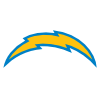Chargers
4.2% implied probability

+2500
25 to 1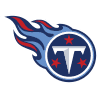Titans
3.4% implied probability

+3500
35 to 1Saints
2.4% implied probability

+5000
50 to 1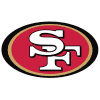49ers
1.7% implied probability

+8000
80 to 1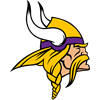Vikings
1.1% implied probability

+10000
100 to 1Patriots
0.9% implied probability

+10000
100 to 1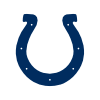Colts
0.9% implied probability

+10000
100 to 1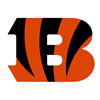Bengals
0.9% implied probability

+10000
100 to 1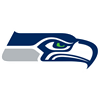Seahawks
0.9% implied probability

+10000
100 to 1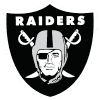Raiders
0.9% implied probability

+10000
100 to 1Steelers
0.9% implied probability

+10000
100 to 1Broncos
0.9% implied probability

+20000
200 to 1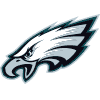Eagles
0.4% implied probability

+20000
200 to 1Panthers
0.4% implied probability

+20000
200 to 1Football Team
0.4% implied probability

+20000
200 to 1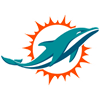Dolphins
0.4% implied probability

+20000
200 to 1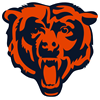Bears
0.4% implied probability

+25000
250 to 1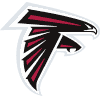Falcons
0.3% implied probability

+50000
500 to 1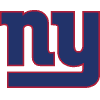Giants
0.2% implied probability

+100000
1000 to 1Jets
0.1% implied probability

+100000
1000 to 1Jaguars
0.1% implied probability

+100000
1000 to 1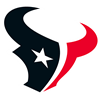Texans
0.1% implied probability

+100000
1000 to 1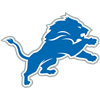Lions
0.1% implied probability